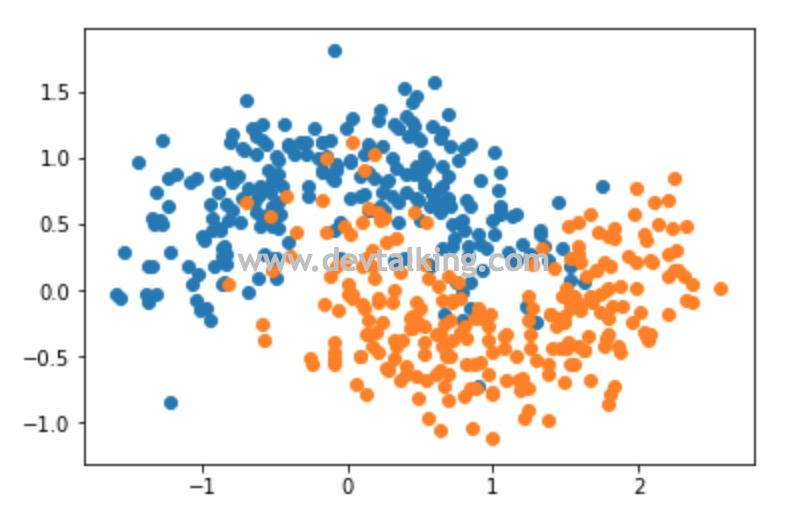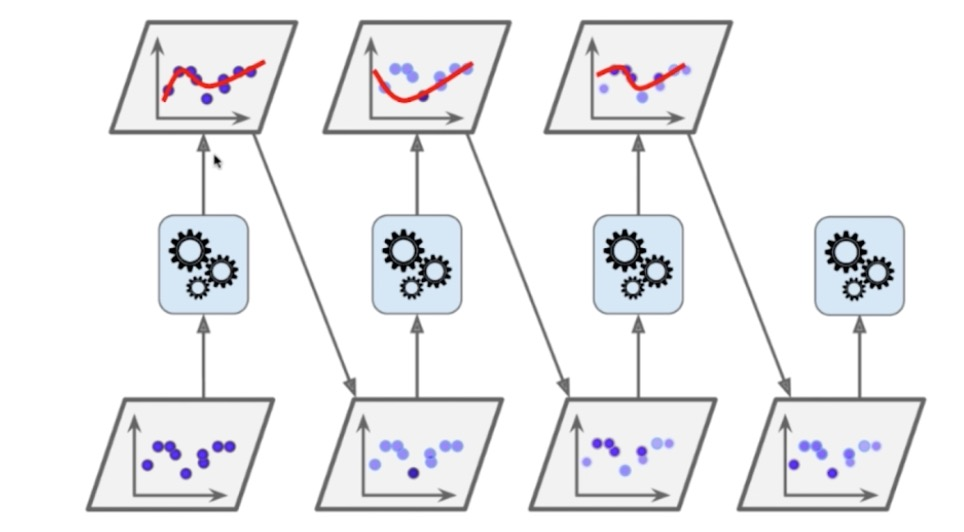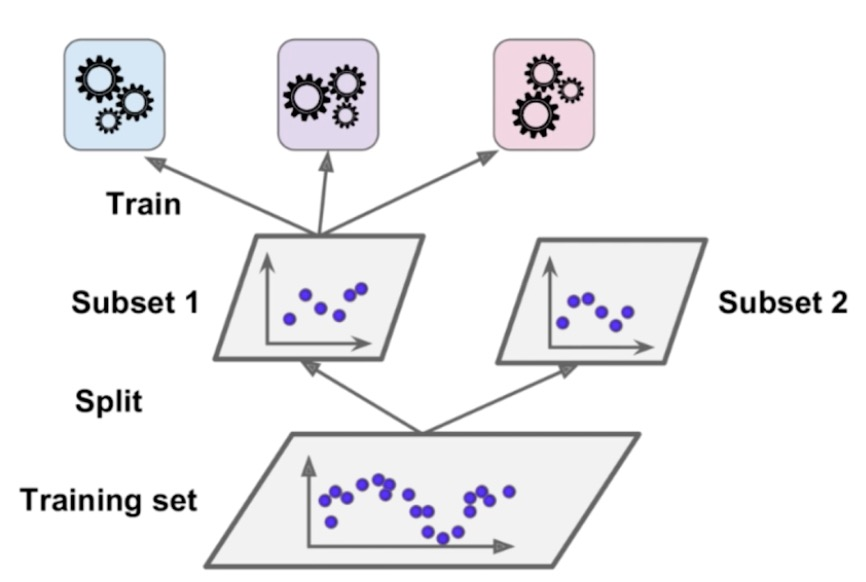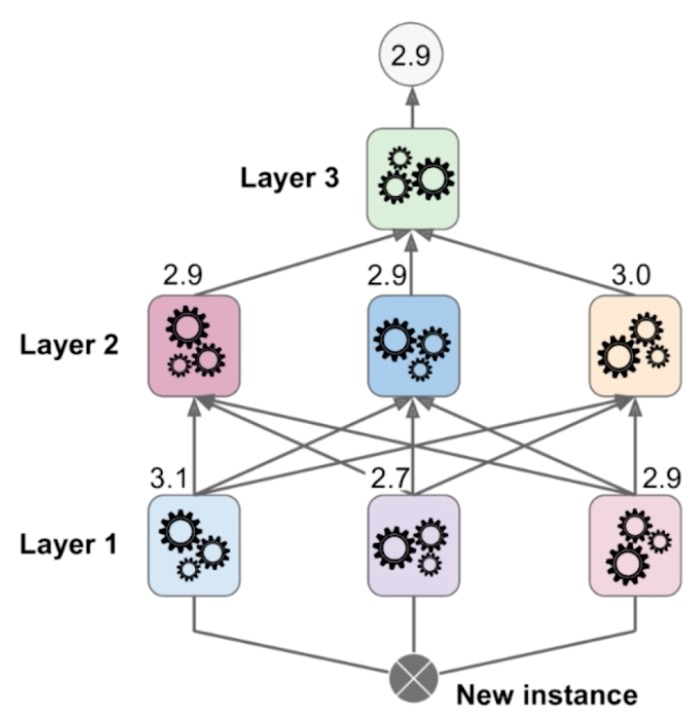集成学习Soft Voting

• 模型1 A-99%，B-1%
• 模型2 A-49%，B-51%
• 模型3 A-40%，B-60%
• 模型4 A-90%，B-10%
• 模型5 A-30%，B-70%

$$\frac {(0.99 + 0.49 + 0.4 + 0.9 + 0.3)} 5 = 0.616$$

$$\frac {(0.01 + 0.51 + 0.6 + 0.1 + 0.7)} 5 = 0.384$$

• 逻辑回归算法本身就是基于概率模型的，通过Sigmoid函数计算概率。
• kNN算法也是支持估计概率的，如果和预测点相邻的3个点，有2个点是红色，1个是蓝色，那么可以很容计算出红色类别的概率是$\frac 2 3$，蓝色类别的概率是$\frac 1 3$。
• 决策树算法也是支持估计概率的，它的思路和kNN的很相近，每个叶子节点中如果信息熵或基尼系数不为0，那么就肯定至少包含2种以上的类别，那么用一个类别的数量除以所有类别的数据就能得到概率。
• SVM算法本身是不能够天然支持估计概率的。不过Scikit Learn中提供的SVC通过其他方式实现了估计概率的能力，代价就是增加了算法的时间复杂度和训练时间。它有一个probability参数，默认为false既不支持估计概率，如果显示传入true，那么就会启用估计概率的能力。

Bagging

$$0.51^3 + C_3^2 \cdot 0.51^2 \cdot 0.49 = 0.515$$

$$\sum_{i=251}^{500}C_{500}^i \cdot 0.51^i \cdot 0.49^{500-i} = 0.656$$

子模型取样方式

• 放回取样：每次取完训练子模型的部分样本数据后，再放回样本数据池里，训练下一个子模型使用同样的方式。这样的方式，训练不同的子模型会可能会用到小部分相同的样本数据。
• 不放回取样：每次取完训练子模型的部分样本数据后，这部分样本数据不再放回样本数据池里，训练下一个子模型使用同样的方式。这样的方式，训练不同的子模型的样本数据不会重复。

随机森林

fit之后，从返回结果里可以看到，RandomForestClassifier的参数综合了BaggingClassifierDecisionTreeClassifier的参数。我们可以对不同的参数进行调优，训练出更好的模型。

BoostingStacking